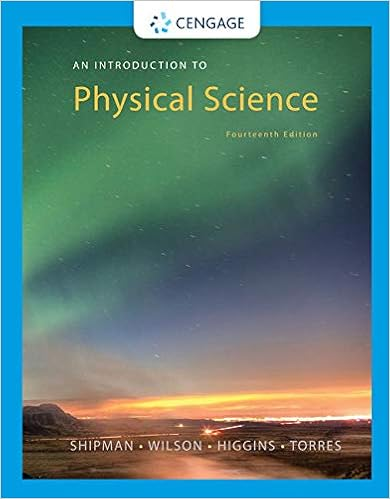# What is the total power dissipated in the two

• Notes
• apiccirello
• 14
• 83% (6) 5 out of 6 people found this document helpful

This preview shows page 6 - 10 out of 14 pages.

##### We have textbook solutions for you!
The document you are viewing contains questions related to this textbook.The document you are viewing contains questions related to this textbook.
Chapter 8 / Exercise 8
An Introduction to Physical Science
Shipman/WilsonExpert Verified
42. What is the total power dissipated in the two resistors in the circuit shown?A) 10 WB) 15 WC) 33 WD) 67 WE) 670 W
43. Three resistors, 4.0-Ω, 8.0-Ω, 16-Ω, are connected in parallel in a circuit. What is the equivalent resistance of this combination of resistors?
44. Five resistors are connected as shown. What is the equivalent resistance between points Aand B?
45. Jason's circuit has a 24-W resistor that is connected in series to two 12-W resistors that are connected in parallel. JoAnna's circuit has three identical resistors wired in parallel. If the equivalent resistance of Jason's circuit is the same as that of JoAnna's circuit, determine the value of JoAnna's resistors.
##### We have textbook solutions for you!
The document you are viewing contains questions related to this textbook.The document you are viewing contains questions related to this textbook.
Chapter 8 / Exercise 8
An Introduction to Physical Science
Shipman/WilsonExpert Verified
Three resistors are connected as shown in the figure below. The potential difference between points Aand Bis 26 V.46. What is the equivalent resistance between the points Aand B?A) 3.8 WB) 4.3 WC) 5.1 WD) 6.8 WE) 9.0 W
47. How much current flows through the 3-W resistor?
48. How much current flows through the 2-W resistor?
Four resistors and a 6-V battery are arranged as shown in the circuit diagram.49. Determine the equivalent resistance for this circuit.
50. The smallest current passes through which resistor(s)?A) the 10-W resistorB) the 20-W resistorC) the 30-W resistorD) the 60-W resistorE) It is the same and the smallest in the 30-W and 60-W resistors.
51. The largest potential difference is across which resistor(s)?
Three resistors are placed in a circuit as shown. The potential difference between points Aand Bis 30 V.52. What is the equivalent resistance between the points Aand B?
53. What is the potential drop across the 10-W resistor?
54. What is the potential drop across the 30-W resistor?A) 10 VB) 20 VC) 30 VD) 60 VE) 100 V
55. What is the current through the 30-W resistor?
56. A non-ideal battery has a 6.0-V emfand an internal resistance of 0.6 Ω. Determine the terminal voltage when the current drawn from the battery is 0.5 A.
57. A battery has a terminal voltage of 12 V when no current flows and an internal resistance of 2 Ω. The battery is placed in series with a 1-Ω resistor. Which one of the entries in the following table is correct?Terminal voltageCurrent through the 1-Ω resistor
12 V4 AD) 12 V12 AE) 18 V3 A
•••